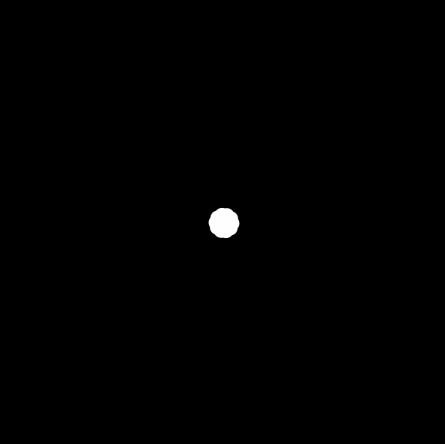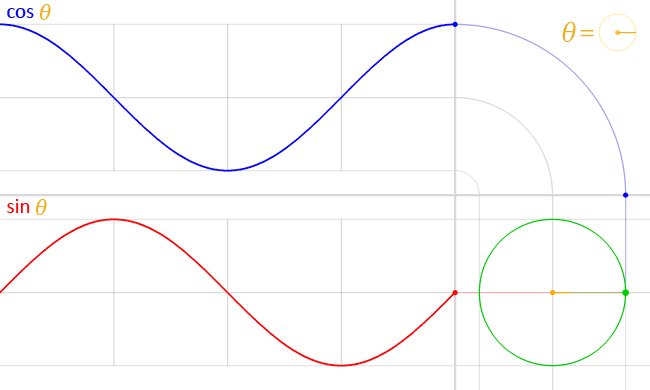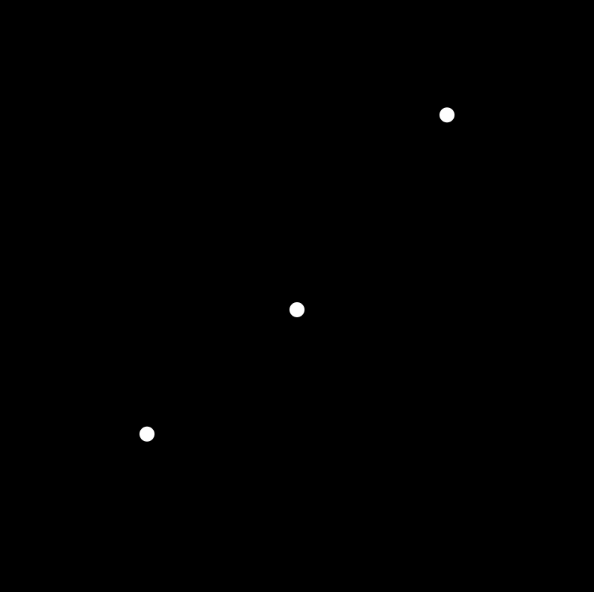# p5: Trigonometric functions and oscillation (sin, cos)

Let’s refresh some basic trigonometry. Sine and cosine are particularly useful when you have to find some x,y coordinates starting from an angle.Here’s how to use the to position things in a circle:```var numObjects = 10;
var centerX;
var centerY;
var distance = 100;

function setup() {
createCanvas(300, 300);
centerX = width / 2;
centerY = height / 2;
noStroke();
ellipseMode(CENTER);
}

function draw() {
background(0);

//divide the circle 360 degrees according to the number of objects
var angleObject = 360 / numObjects;

for (var i = 0; i < numObjects; i++) {

//the sin(angle) cos(angle) has to be
//multiplied by the distance from the center
//because trigonometric functions assume a circle with radius=1
//centerX and centerY is the offset from the top left corner
//remember to convert in radians
var posX = centerX + distance * cos(radians(angleObject * i ));
var posY = centerY + distance * sin(radians(angleObject * i ));
fill(255);
ellipse(posX, posY, 10, 10);
}
}
```

Make it rotate without using the transformations
Make it draw a pentagon
Make it draw a star
Make the distance from the center oscillate harmonically (see oscillation below)Here’s how to obtain the same result using the transform functions we covered last time

```var numObjects = 10;
var centerX;
var centerY;
var distance = 100;

function setup() {
createCanvas(600, 600);
centerX = width / 2;
centerY = height / 2;

noStroke();
ellipseMode(CENTER);
}

function draw() {
background(0);

var angleObject = 360 / numObjects;
for (var i = 0; i < numObjects; i++) {

push();
translate(centerX, centerY);
rotate(radians(i * angleObject));
//or you can draw at 0,0 and translate again
//translate(distance,0);
ellipse(distance, 0, 10, 10);
pop();
}
}```

## Oscillations

Trigonometric functions can be used to create harmonic oscillationsExample comparing the uses of noise and trigonometric functions (sin) for animation```var a = 0;
var n = 0;
var r = 0;

function setup() {
createCanvas(400, 400);
}

function draw() {
background(0);
fill(255);
noStroke();

///////////////////
//harmonic oscillation
//I increase an angle by one degree each frame
//and calculate the sine
//sine is a number between -1 and 1 so I need to multiply it
a += 1;
var ySin = sin(radians(a)) * 100;
//adding the sine to y starting from the center position
ellipse(100, 200 + ySin, 10, 10);

/////////////////
//Perlin noise (one dimensional)
//noise requires a number that increases linearly
n += 0.01;

//I use this n as single parameter to generate
//the noise value at that "position" in the noise landscape
//since noise is 0 to 1 I subtract 0.5 to make is equally
//balanced between negative and positive values

var yNoise = (noise(n) -0.5) * 100;
ellipse(200, 200 + yNoise, 10, 10);

////////////////////
//random walk: each time I add a random value
//noise and sin circles will be move in the same at each run
//random circle will not
r += random(-5, 5);
ellipse(300, 200 + r, 10, 10);
}```